StatLect

# Event

An event is a subset of the set of all possible outcomes of a probabilistic experiment.

The concept of event is fundamental in probability theory. In fact, whenever we speak about probability, we explicitly or implicitly refer to the probability of an event.## Set notation

The set of all possible outcomes is called sample space and it is usually denoted by.

Thus, an eventis a subset of, which is indicated bywhereis the inclusion symbol.

## Example

If the probabilistic experiment is the roll of a die, the set of all possible outcomes is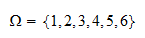In this setting, three instances of events are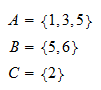The eventcould be described in words as "An odd number appears face up".

The eventcould be described as "A number strictly greater than four appears face up".

The eventcould be described as "The numberappears face up".

In case you are wondering about the curly-bracket notation, you are strongly advised to revise the introductory lecture on set theory, where we explain how the notation is used.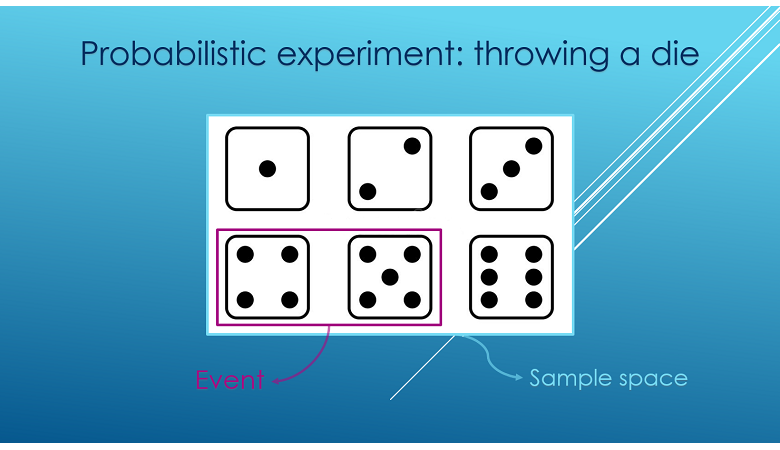## Probability of an event

In a probabilistic model, each eventis assigned a probability, which measures how likely it is thatwill happen.

Example If, as in the above example, the probabilistic experiment is the roll of a die, we could have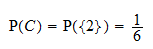and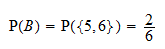In other words, the probabilities of the eventsandare one and two sixths, respectively.

## Special events

There are some events that have special names.

### Sure event

A sure event is an event that always happens. It coincides with the set of all possible outcomes.

If we employ the same notation used above,is the sure event.

In the roll-of-a-die example, the sure eventcould be described in words as "Any one of the numbers from 1 to 6 appears face up".

### Impossible event

An impossible event is an event that never happens. It coincides with the empty set.

You can find a careful discussion of this apparently strange definition in the glossary entry on impossible events.

## Union of events

When we compute probabilities, we often deal with the union of two or more events.

The union of two eventsandis itself an event. It comprises all the outcomes that belong toor to.

In the above example, we had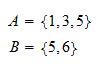Then, the union ofand, denoted by, is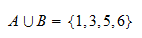## Intersection of events

The intersection ofandis the event that comprises all the outcomes that belong both toand to.

Ifandare defined as above, then the intersection ofand, denoted by, is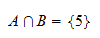## Mutually exclusive events

Two events are said to be mutually exclusive if their intersection is equal to the empty set.

For example,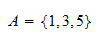andare mutually exclusive because## Events as measurable sets

In simple probabilistic settings, all subsets of the sample spaceare considered events. However, there are also more complicated settings in which some subsets ofcannot be considered events. In those complicated settings, the sets that can be considered events are called measurable sets (see the lecture on Probability).

## More details

For other examples and more detailed explanations of the concept of event, you can go to the lecture entitled Probability.

Moreover, you might be interested in the following more advanced concepts:

Previous entry: Estimator

Next entry: Factorial